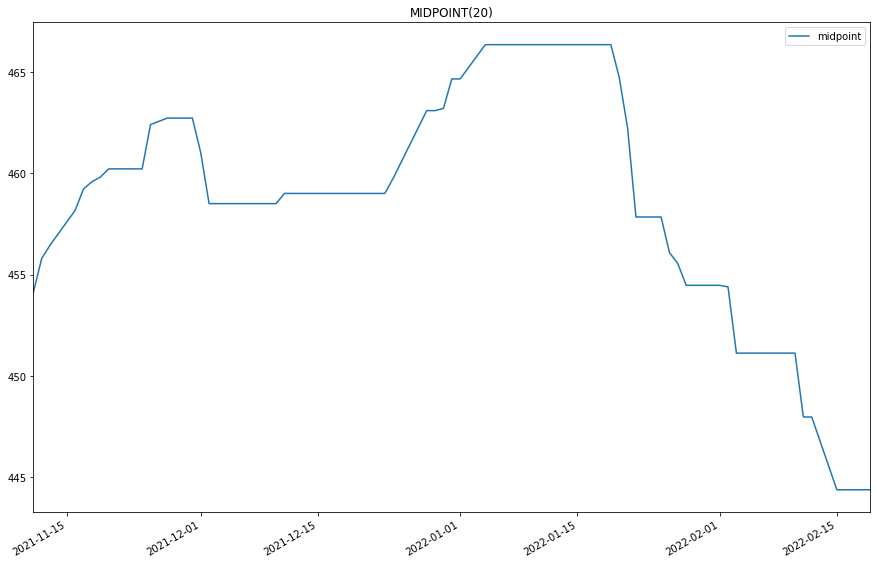# Indicator Reference

## Mid Point

### Introduction

This indicator computes the MidPoint (MIDPOINT) The MidPoint is calculated using the following formula: MIDPOINT = (Highest Value + Lowest Value) / 2

### Create Manual Indicators

You can manually create a MidPoint indicator, so it doesn’t automatically update. Manual indicators let you update their values with any data you choose. The following reference table describes the MidPoint constructor.

### MidPoint()1/2

            MidPoint QuantConnect.Indicators.MidPoint (
string  name,
int     period
)


Initializes a new instance of the MidPoint class using the specified name and period.

### MidPoint()2/2

            MidPoint QuantConnect.Indicators.MidPoint (
int  period
)


Initializes a new instance of the MidPoint class using the specified period.

### Update Manual Indicators

You can update the indicator automatically or manually.

#### Automatic Update

To register a manual indicator for automatic updates with the security data, call the RegisterIndicator method.


private MidPoint _midpoint;

// In Initialize()
_midpoint = new MidPoint(name, period);
_midpoint.Updated += IndicatorUpdateMethod;

RegisterIndicator(symbol, _midpoint, Resolution.Daily);

// In IndicatorUpdateMethod()
{
var indicatorValue = _midpoint.Current.Value;
}

# In Initialize()
self.midpoint = MidPoint(name, period)
self.midpoint.Updated += self.IndicatorUpdateMethod

self.RegisterIndicator(symbol, self.midpoint, Resolution.Daily)

# In IndicatorUpdateMethod()
indicator_value = self.midpoint.Current.Value

To customize the data that automatically updates the indicator, see Custom Indicator Periods and Custom Indicator Values.

#### Manual Update

Updating your indicator manually enables you to control when the indicator is updated and what data you use to update it. To manually update the indicator, call the Update method with time/decimal pair. The indicator will only be ready after you prime it with enough data.


private MidPoint _midpoint;
private Symbol symbol;

// In Initialize()
_midpoint = new MidPoint(period);

// In OnData()
if (data.ContainsKey(_symbol))
{
_midpoint.Update(data[symbol].EndTime, data[symbol].High);
}
{
var indicatorValue = _midpoint.Current.Value;
}

# In Initialize()
self.midpoint = MidPoint(period)

# In OnData()
if data.ContainsKey(self.symbol):
self.midpoint.Update(data[self.symbol].EndTime, data[self.symbol].High)
indicator_value = self.midpoint.Current.Value

### Create Automatic Indicators

The MIDPOINT method creates an MidPoint indicator, sets up a consolidator to update the indicator, and then returns the indicator so you can use it in your algorithm.

The following reference table describes the MIDPOINT method:

### MIDPOINT()1/1

            MidPoint QuantConnect.Algorithm.QCAlgorithm.MIDPOINT (
Symbol                           symbol,
Int32                            period,
*Nullable<Resolution>      resolution,
*Func<IBaseData, Decimal>  selector
)


Creates a new MidPoint indicator.

If you don't provide a resolution, it defauls to the security resolution. If you provide a resolution, it must be greater than or equal to the resolution of the security. For instance, if you subscribe to hourly data for a security, you should update its indicator with data that spans 1 hour or longer.

### Get Indicator Values

To get the value of the indicator, use its Current.Value attribute.

private MidPoint _midpoint;

// In Initialize()
_midpoint = MIDPOINT(symbol, period);

// In OnData()
{
var current = _midpoint.Current.Value;
}
# In Initialize()
self.midpoint = self.MIDPOINT(symbol, period)

# In OnData()
current = self.midpoint.Current.Value


### Visualization

To plot indicator values, in the OnData event handler, call the Plot method.

private MidPoint _midpoint;

// In Initialize()
_midpoint = MIDPOINT(symbol, period);

// In OnData()
{
Plot("My Indicators", "midpoint", _midpoint.Current);
}
# In Initialize()